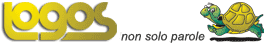Select LanguageKurt Gödel (1906-1978)   Austrian-American mathematician who proved that, if you begin with any sufficiently strong consistent system of axioms, there will always be statements within the system governed by those axioms that can neither be proved or disproved on the basis of those axioms. Hence, it in undecidable on the basis of those axioms whether the system contains paradoxes. The formal statement of this fact is known as Gödel's incompleteness theorem. Gödel also proved Gödel's completeness theorem, which states that if T is a set of axioms in a first-order language, and a statement p holds for any structure M satisfying T, then p can be formally deduced from T in some appropriately defined fashion. Gödel showed that no contradiction would arise if the continuum hypothesis were added to conventional Zermelo-Fraenkel set theory. However, using a technique called forcing, Paul Cohen (1963, 1964) proved that no contradiction would arise if the negation of the continuum hypothesis was added to set theory. Together, Gödel's and Cohen's results established that the validity of the continuum hypothesis depends on the version of set theory being used, and is therefore undecidable (assuming the Zermelo-Fraenkel axioms together with the axiom of choice ).